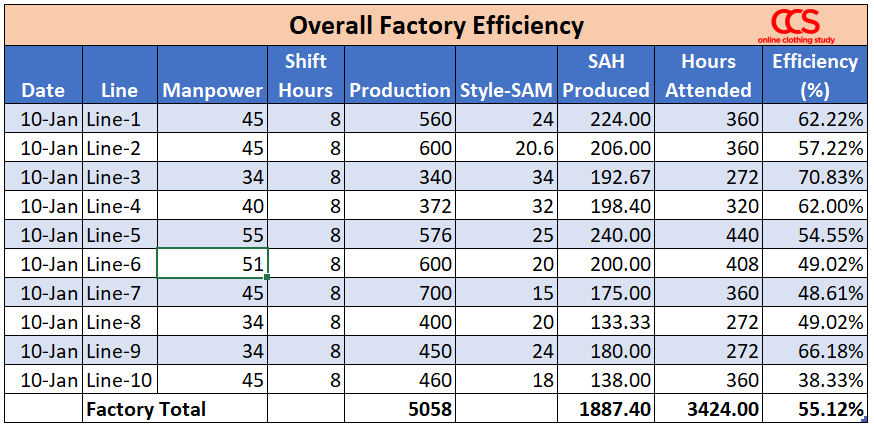# How to calculate daily factory efficiency on an overall basis?

Question: How to calculate daily factory efficiency on an overall basis for a garment factory having 10 production lines? Should we consider removing quality defects % in the calculation?

To answer this question, I will show you a definite guide, how one can calculate daily factory efficiency with an example.

When it comes to calculating the overall efficiency of a garment factory, we need to consider all working hours attended by sewing operators. In practice, there will be some idle time, some non-productive times during the day. We will not deduct any hours (loss time) when calculating factory efficiency overall basis.

Engineers calculate the total SAM produced by a production line from the production quantity (line output). In the article, I will use SAH produced and present SAM produced in hours.

Calculate Total SAH produced by all 10 lines and total Hours Worked by each line.

### #1. Calculate total SAH produced by line

Collect line-wise production quantity, Styles numbers, and Style SAM. From these data, you need to calculate SAH produced by line.

Line SAH = (Line output x Style SAM)/60

In the example, Line-1 has an output of 560 pieces, and style SAM is 24 Minutes.

Therefore, SAH produced by Line-1 is 224 Hours

Note: If some of your lines work on more than one style and you get output from multiple styles from a single line, then follow this.

SAH produced = ((Style-1 output x Style-1 SAM) + (Stye-2 output X Stye-2 SAM) + (Style-3 output x Style-3 SAM))/60.

### #2. Calculate total hours attended (hours worked) by line

Get the line-wise attendance of the operators and helpers from the line supervisors. There will be employee movement from one line to another during the working hours. Make sure that employee headcount is not counted twice in calculating working hours.

Hours worked = (Manpower x Shift Hours)

Normally, factories work for 8 hours shift a day. If your shift hour is different (e.g 9 hours, 10 hours, 11 hours), use that.

In the example, Line-1 has 45 manpower, and it works for 8 hours. Therefore, Total hours worked in Line-1 = (45 x 8) = 360 Hours

### #3. Calculate line efficiency

Individual line efficiency = (Total SAH / Total Hours )*100

For Line-1, line efficiency will be = (224/360)*100 = 62.22%

### #4. Calculate overall factory efficiency

You have hours worked ad SAH data of all sewing lines. Now calculate total hours worked and SAH produced of all 10 lines. See the below example.

Refer to the following table to understand what data you need to collect by line.Calculate Factory Efficiency = (Sum of 10 lines SAH / Sum of 10 lines Total Hours ) * 100

In the above example, you see total production volume of the factory is 5058 pieces. You get the total SAH produced 1887.40 Hours and Total hours worked 3424 Hours. Based on these two data, calculate overall efficiency of the factory.

Overall  factory efficiency = 55.12%

Also Read: Calculating overall efficiency of a production line

Question#2: Should we consider removing quality defects % in the calculation?

At the end-of-line checking if you are segregating defective pieces and good pieces, and only sending the QC passed pieces to the next process, then you should count passed quantity as line’s production.

It solely depends on the factory’s practice whether to consider only QC passed quantity as line's production quantity or all the stitched quantity at the last operations.

### Prasanta Sarkar

Prasanta Sarkar is a textile engineer and a postgraduate in fashion technology from NIFT, New Delhi, India. He has authored 6 books in the field of garment manufacturing technology, garment business setup, and industrial engineering. He loves writing how-to guide articles in the fashion industry niche. He has been working in the apparel manufacturing industry since 2006. He has visited garment factories in many countries and implemented process improvement projects in numerous garment units in different continents including Asia, Europe, and South Africa. He is the founder and editor of the Online Clothing Study Blog. He lives in India. linkedin